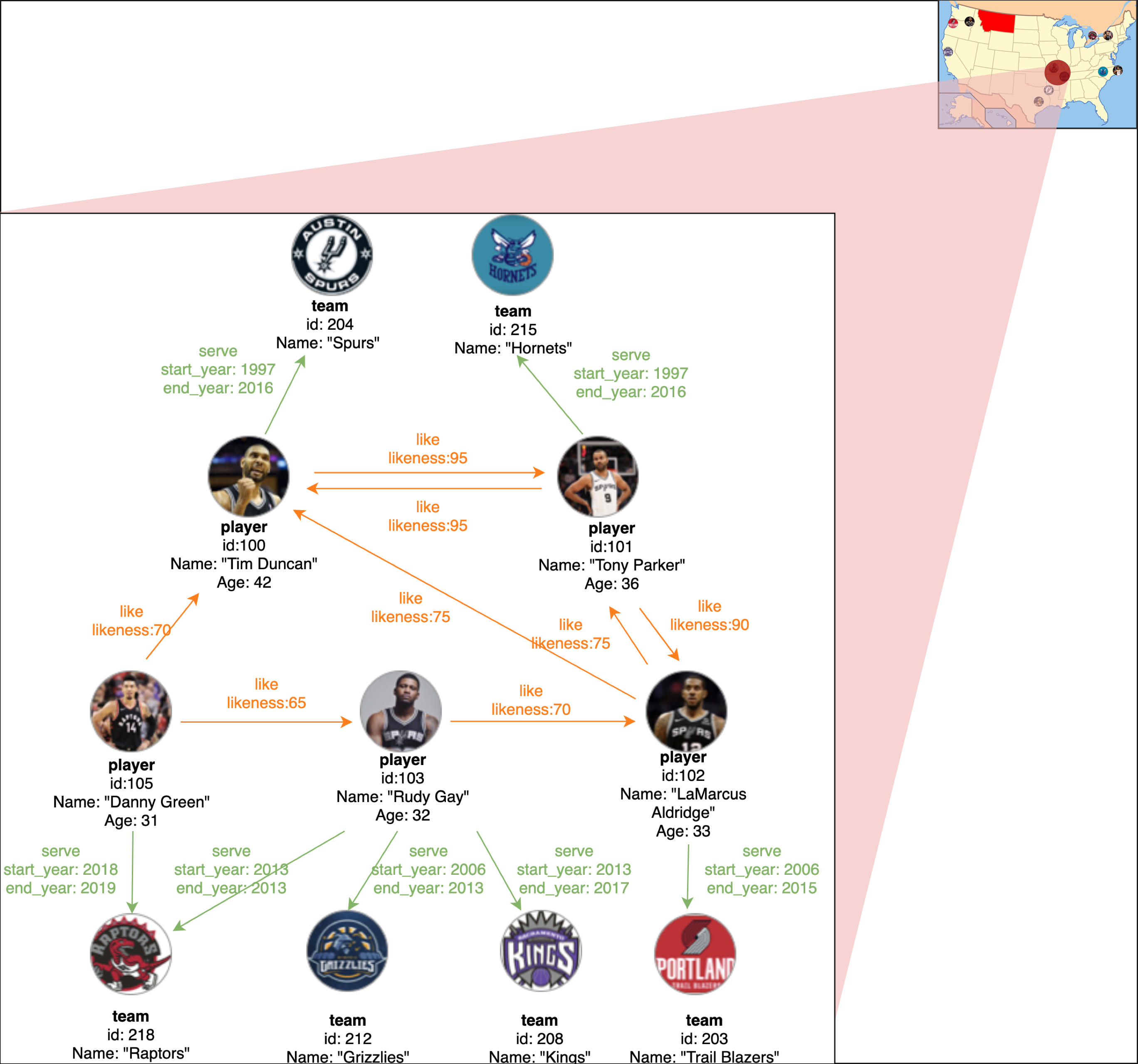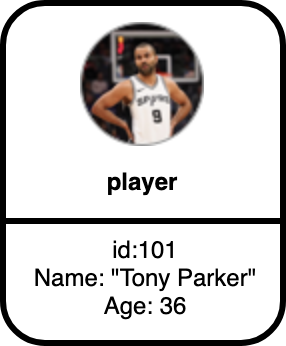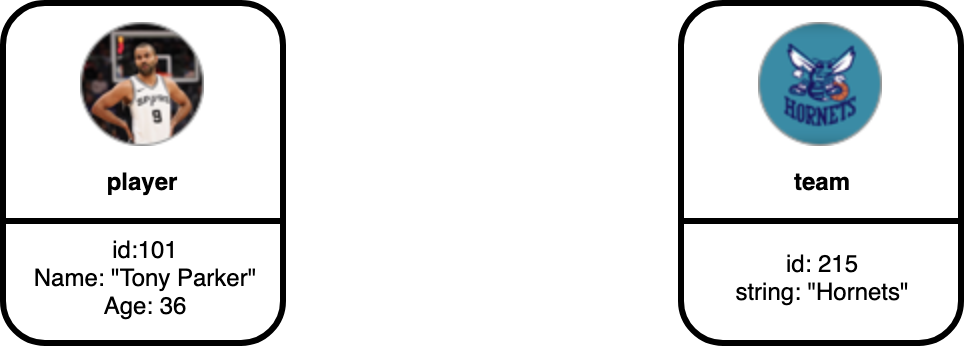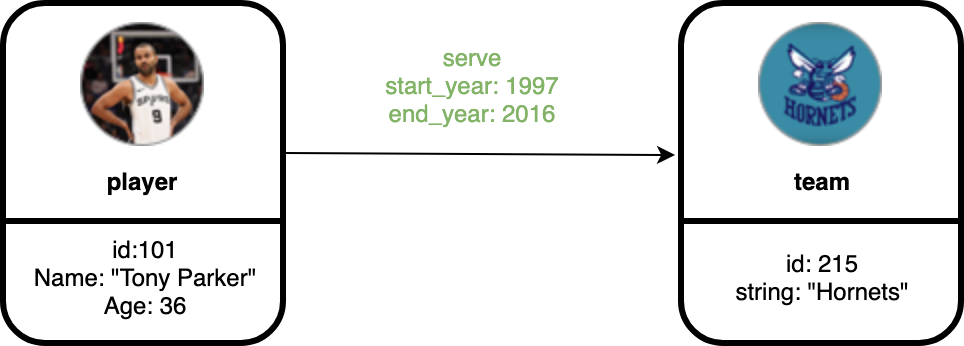Fetching contributors…Cannot retrieve contributors at this time
52 lines (28 sloc) 3.01 KB

# Graph Data Modeling

This guide is designed to walk you through the graph data modeling of Nebula Graph. Basic concepts of designing a graph data model will be introduced.

## Graph Space

Graph Space is a physically isolated space for different graph. It is similar to database in MySQL.

## Directed Property Graph

The data model handled by the Nebula Graph is a directed property graph, whose edges are directional and there could be properties on both edges and vertices. It can be represented as: G = < V, E, PV, PE >, where V is a set of nodes aka vertices, E is a set of directional edges, PV represents properties on vertices, and PE is the properties on edges.

We will use the example graph below to introduce the basic concepts of property graph:In the preceding picture, we have a data set about the players and teams information of NBA. We can see the eleven vertices are classified to two kinds, i.e. player and name while the fifteen edges are classified to serve and like.

To better understand the elements of a graph data model, let us walk through each concept of the example graph.

## Vertices

Vertices are typically used to represent entities in the real world. In the preceding example, the graph contains eleven vertices.## Tags

In Nebula Graph, vertex properties are clustered by tags. In the example above, the vertices have tags player and team.## Edge

Edges are used to connect vertices. Each edge usually represents a relationship or a behavior between two vertices. In the preceding example, edges are serve and like.## Edge Type

`Each edge` is an instance of an edge type. Our example uses serve and like as edge types. Take edge serve for example, in the preceding picture, vertex `101` (represents a player) is the source vertex and vertex `215` (represents a team) is the target vertex. We see that vertex `101` has an outgoing edge while vertex `215` has an incoming edge.

## Properties

Properties are named-value pairs within vertices and edges. In our example graph, we have used the properties `id`, `name` and `age` on player, `id` and `name` on team, and `likeness` on like edge.

## Schema

In Nebula Graph, schema refers to the definition of properties (name, type, etc.). Like `MySQL`, Nebula Graph is a strong typed database. The name and data type of the properties should be determined before the data is written.

You can’t perform that action at this time.